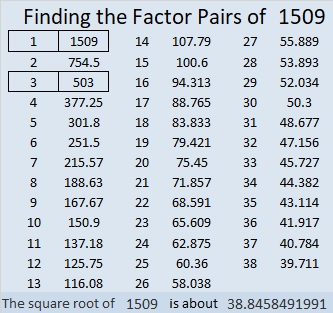# 1509 A Mystery Level Puzzle on Training Wheels

Contents

### Today’s Puzzle:

I have described level 3 puzzles as level 4 puzzles on training wheels. Today’s puzzle is definitely not a level 3 puzzle, but it is on training wheels. A logical way to solve this puzzle is to start with the clue in the top row and work your way down the puzzle row by row writing the factors as you go. Giving you the logical order to use the clues should help some, but the logic needed to find the factors will still be a mystery. Don’t guess and check. Please, use logic! Think about how each clue relates to the other clues in the puzzle.### Factors of 1509:

• 1509 is a composite number.
• Prime factorization: 1509 = 3 × 503.
• 1509 has no exponents greater than 1 in its prime factorization, so √1509 cannot be simplified.
• The exponents in the prime factorization are 1, and 1. Adding one to each exponent and multiplying we get (1 + 1)(1 + 1) = 2 × 2 = 4. Therefore 1509 has exactly 4 factors.
• The factors of 1509 are outlined with their factor pair partners in the graphic below.### More about the Number 1509:

1509 is the difference of two squares in two different ways:
755² – 754² = 1509
253² – 250² = 1509

## One thought on “1509 A Mystery Level Puzzle on Training Wheels”

1.ivasallay

Here’s a hint to help you deal with the 16. Is it 2 × 8 or 4 × 4? The other clues in the puzzle leave only one possibility.

Now to deal with the 20, there are only two clues in the puzzle that are divisible by 5 or 10. That means that the cell above the blank column has to be either a 5 or 10. Whichever one it is, the cell above the 50 will be the other one. You don’t need to know which is which to figure out where to put the factors of 20. Also, the other clues in the puzzle will let you know if 20 is 2 × 10 or 4 × 5.

This site uses Akismet to reduce spam. Learn how your comment data is processed.